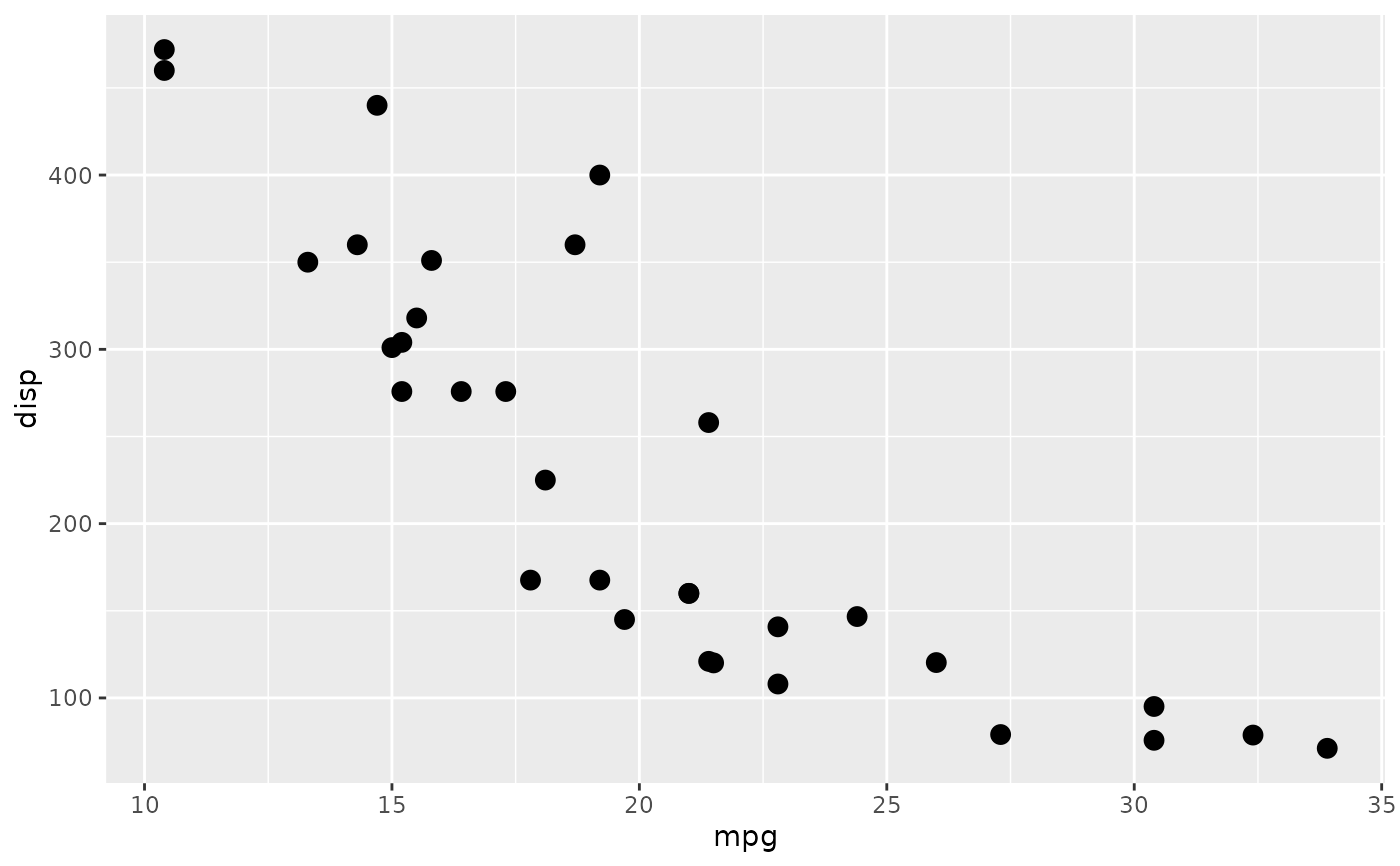This filter simply converts the given layer, grob, or ggplot to a raster and inserts it back again. It is useful for vector graphics devices such as svglite if a layer contains a huge amount of primitives that would make the file slow to render. as_reference(x, id) is a shorthand for with_raster(x, id = id, include = FALSE) that makes the intent of using this grob or layer as only a filter reference clear.

with_raster(x, ...)

## Arguments

x

A ggplot2 layer object, a ggplot, a grob, or a character string naming a filter

...

Arguments to be passed on to methods. See the documentation of supported object for a description of object specific arguments.

## Value

Depending on the input, either a grob, Layer, list of Layers, guide, or element object. Assume the output can be used in the same context as the input.

## Examples

library(ggplot2)
ggplot(mtcars, aes(mpg, disp)) +
with_raster(geom_point(data = mtcars, size = 3))